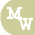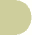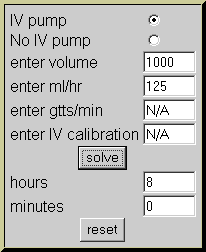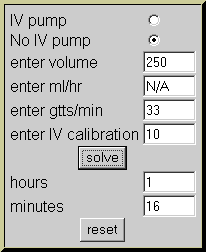IV infusion timenursing calculators search site map

 IV pump No IV pump enter volume enter ml/hr enter gtts/min enter IV calibration hours minutes

Formulas

Basic Time

 Volume Rate = Hours

Time Calculated Using IV Tubing Only

 Volume / ( gtt/min  Calibration x  60 ) = Hours

Examples

1. Using a volumetric IV pump calculate to duration of 1000 mL of normal saline infusing at 125mL/hour.

select "IV pump"
volume = 1000 milliliters
rate = 125 mL/hr

Actual formula:

 1000mL 125 = 8 Hours2. There are 250 mL of D5W infusing at 33 gtt/min on IV tubing calibrated at 10 gtt/mL.  Calculate the infusion time.

select "No IV pump"
volume = 250 mL
rate = 33 gtt/min
calibration = 10 gtt/mL

Actual formula:

 250 / ( 33 gtt/min 10 x  60 ) = 1.26 Hours

0.26 x 60 = 16 minutes
So 1.26 Hours = 1 Hour & 16 minutes